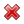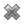# Fight Finance

#### CoursesTagsRandomAllRecentScoresScoreskeithphw $6,011.61 Jade$1,815.80 Chu $789.98 royal ne...$750.00 Leehy $713.33 Visitor$650.00 JennyLI $625.61 Visitor$590.00 ZOE HY $560.00 Visitor$555.33 Visitor $550.00 Visitor$550.00 Visitor $540.00 Visitor$500.00 Yizhou $489.18 Visitor$480.00 Visitor $464.70 Visitor$460.00 LWH $460.00 Visitor$460.00

A risk manager has identified that their hedge fund’s continuously compounded portfolio returns are normally distributed with a mean of 10% pa and a standard deviation of 30% pa. The hedge fund’s portfolio is currently valued at $100 million. Assume that there is no estimation error in these figures and that the normal cumulative density function at 1.644853627 is 95%. Which of the following statements is NOT correct? All answers are rounded to the nearest dollar. A risk manager has identified that their pension fund’s continuously compounded portfolio returns are normally distributed with a mean of 5% pa and a standard deviation of 20% pa. The fund’s portfolio is currently valued at$1 million. Assume that there is no estimation error in the above figures. To simplify your calculations, all answers below use 2.33 as an approximation for the normal inverse cumulative density function at 99%. All answers are rounded to the nearest dollar. Which of the following statements is NOT correct?

A company has a 95% daily Value at Risk (VaR) of \$1 million. The units of this VaR are in:

The 95% daily VaR corresponds to the result on the: